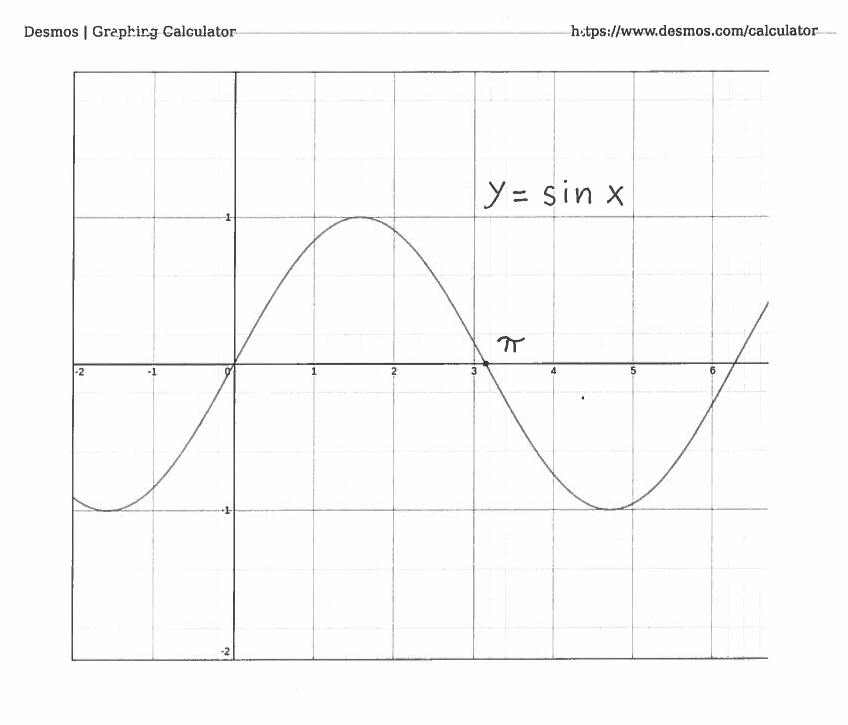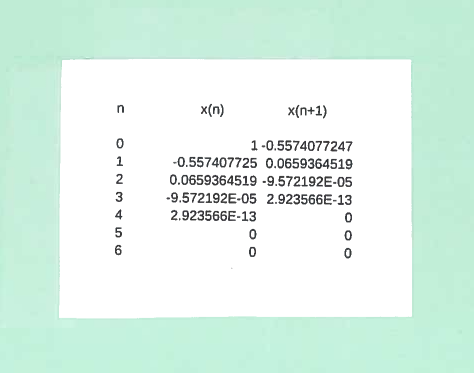SOLUTION 4: We know from trigonometry that $\ \sin \pi = 0$, so I will choose to solve the equation $\ \sin x = 0 \$ for $x$. Let's define function $f(x) = \sin x$, whose graph is given below.The derivative of $f$ is $f'(x) = \cos x$. Now use Newton's Method: $$x_{n+1} = x_{n} - { f(x_{n}) \over f'(x_{n}) } \ \ \ \ \longrightarrow$$ $$x_{n+1} = x_{n} - { \sin x_{n} \over \cos x_{n} } \ \ \ \ \longrightarrow$$ $$x_{n+1} = x_{n} - { \tan x_{n} }$$ I will choose to let $x_{0}=2$. Using Newton's Method formula for 7 iterations in a spreadsheet results in :Thus $\ \pi \$ to ten decimal places is $\ \pi \approx 3.1415926536$.

IMPORTANT NOTE: Choosing a different initial guess can lead to other solutions to the equation $\ \sin x = 0 \$. For example, if I choose to let $x_{0}= 1$, then Newton's Method leads to the solution $\ x=0 \$. Using Newton's Method formula for iterations in a spreadsheet results in :